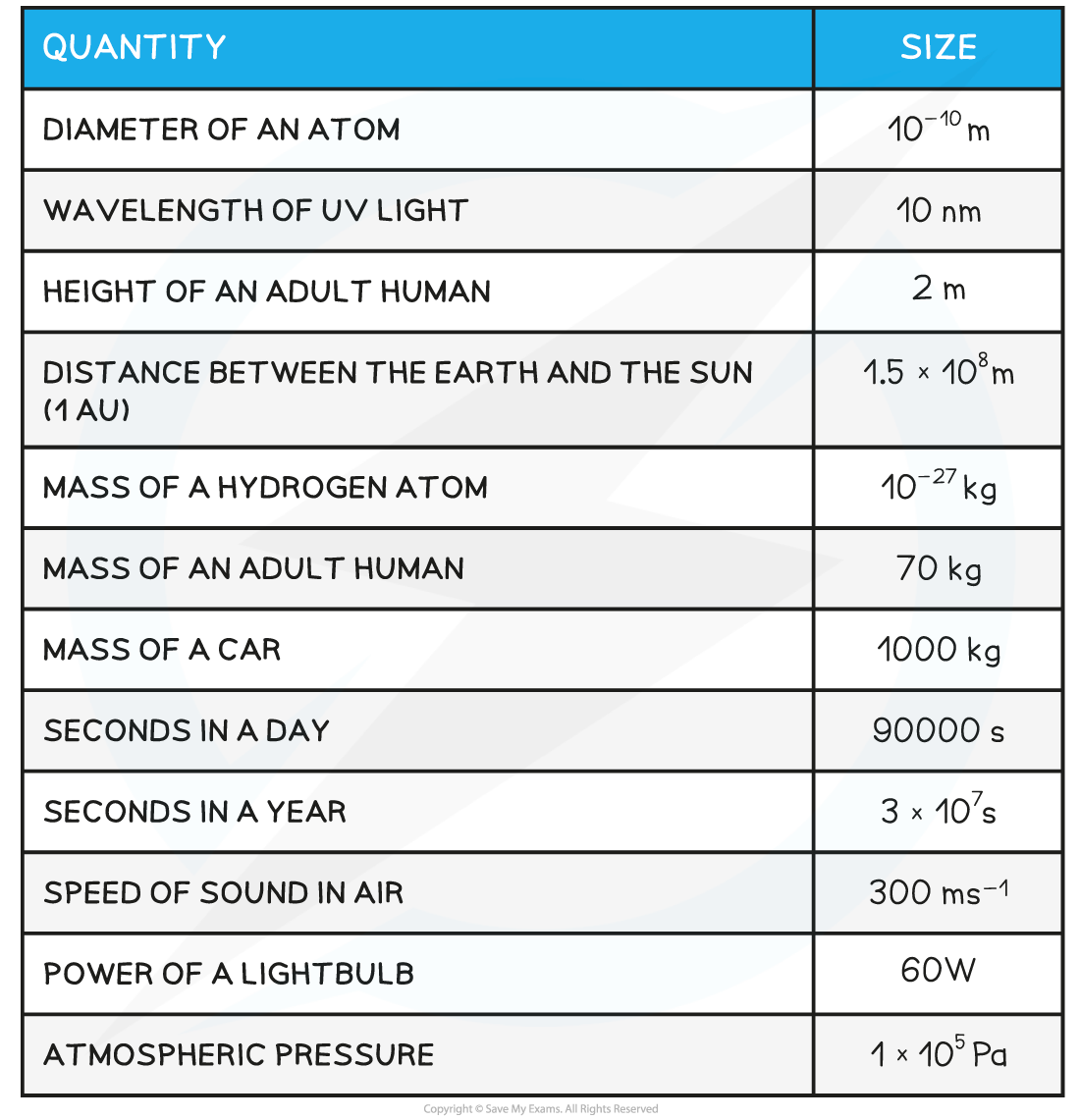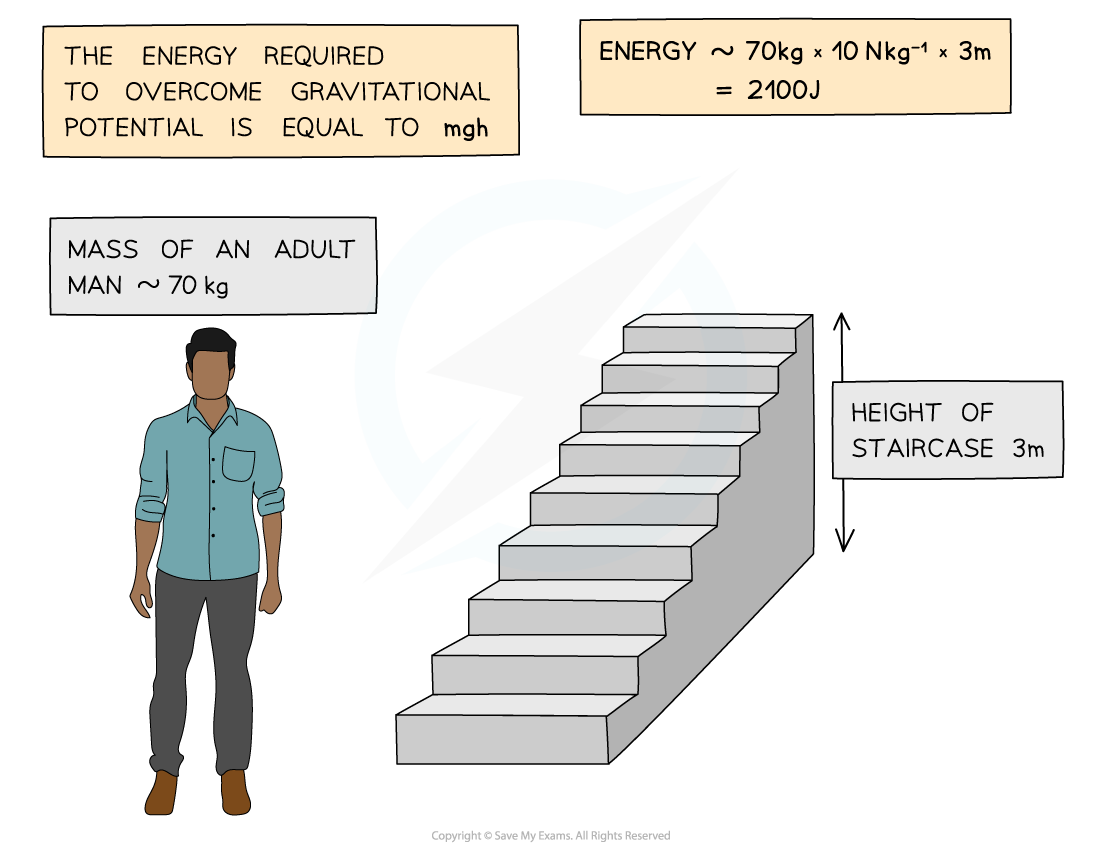# Edexcel A Level Physics:复习笔记1.3 Estimating Physical Quantities

### Estimate Physical Quantities

• There are important physical quantities to learn in physics
• They are particularly useful when making estimates
• A few examples of useful quantities to memorise are given in the table below (this is by no means an exhaustive list)

Estimating Physical Quantities Table#### Worked Example

Estimate the energy required for an adult man to walk up a flight of stairs.#### Exam Tip

The mark scheme for calculations involving estimates are normally quite generous and offer a range of values as the final answer. Some common estimates are:

• Mass of an adult = 70 kg
• Gravitational field strength, g = 10 m s-2
• Mass of a car = 1500 kg
• Wavelength of visible light = 400 nm (violet) – 700 nm (red)

Many values are already given in your data booklet that therefore may not be given in the question, so make sure to check there too!